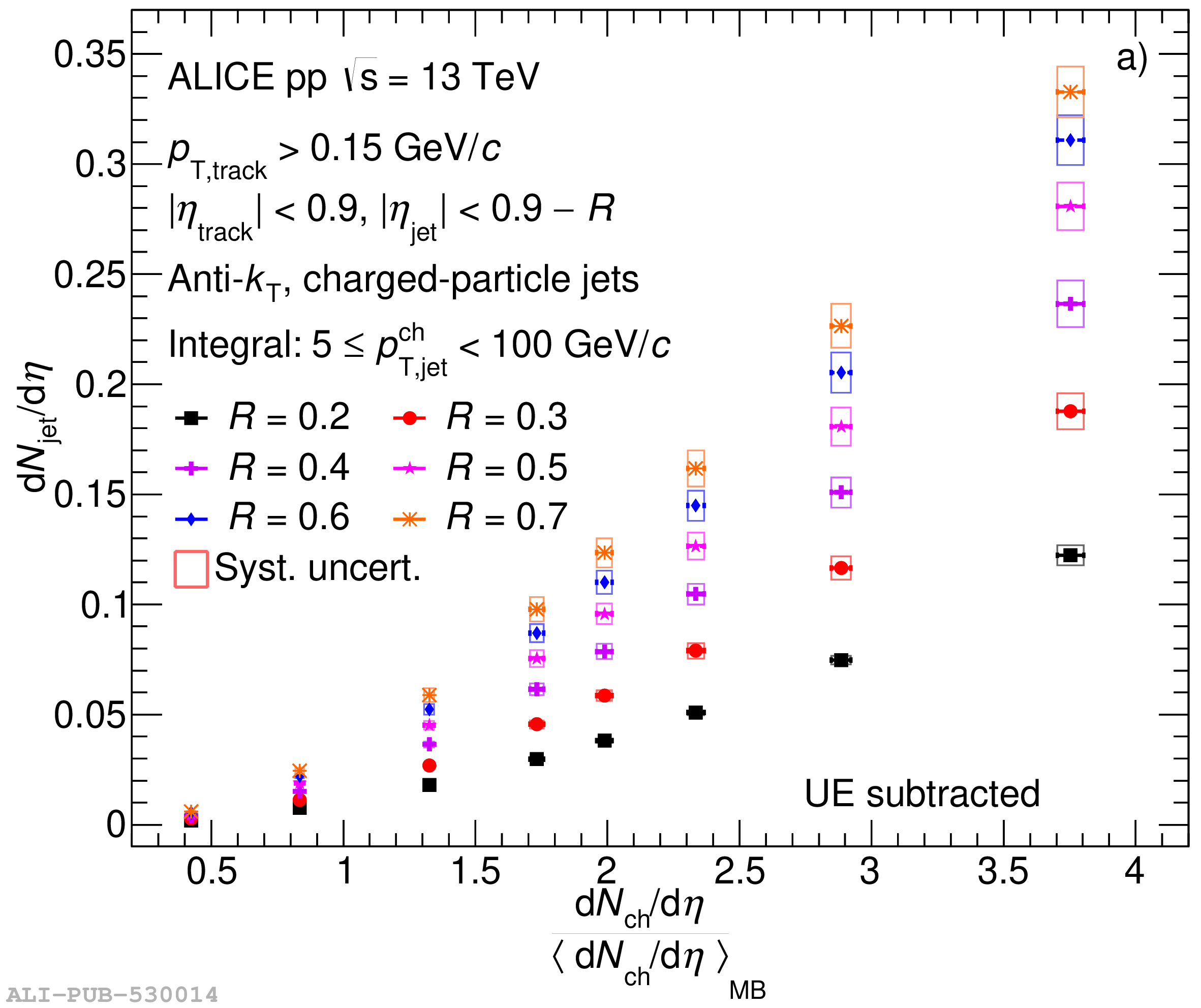# Figure 12

 a) Integrated jet yields, and b) $\left \langle p_{\rm T} \right \rangle$ of jets with $5\leq p_{\rm T,jet}^{\rm ch}< 100\ {\rm GeV}/c$ as a function of self-normalised charged-particle multiplicity for different resolution parameters $R$ varied from $0.2$ to $0.7$, with the charged-particle multiplicities provided in Ref. . Statistical and systematic uncertainties are shown as vertical error bars and boxes around the data points, respectively.